# 第四次作业

## 1.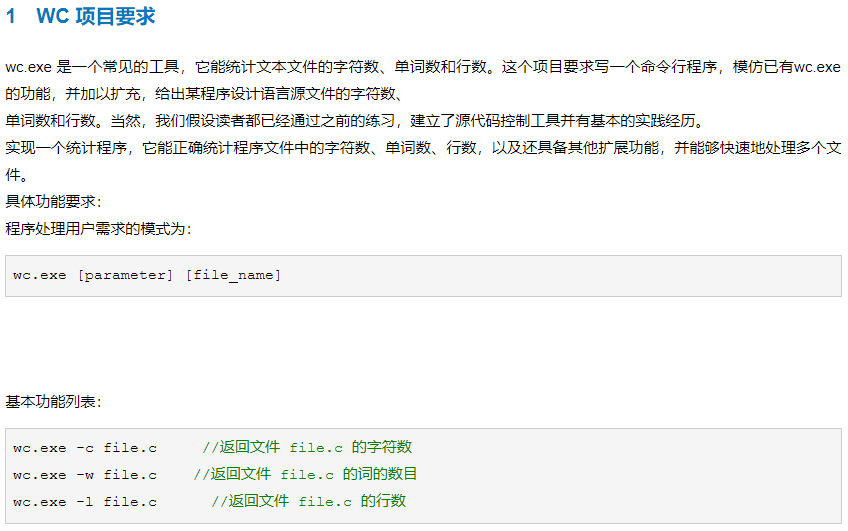### 2.代码：

#include <stdio.h>
#include <stdlib.h>
#include <string.h>
int zifushu(FILE *fp);
int cishu(FILE *fp);
int line(FILE *fp);
int main(int argc,char *argv[])
{
FILE *fp;
if((fp=fopen("file.c","r"))==NULL){
printf("cannot open file\n");
exit(0);
}
char a;
int count;
scanf("%c",&a);
if(a=='c'){
count=zifushu(fp);
}else if(a=='w'){
count=cishu(fp);
}else if(a=='l'){
count=line(fp);
}
printf("%d",count);
fclose(fp);
return 0;
}
int zifushu(FILE *fp){
/*FILE *fp;
if(fp=fopen(file.c,"r")==NULL){
printf("cannot open file\n");
exit(0);
}*/
char c;
int count=0;
while(!feof(fp)){
c=fgetc(fp);
if(c>=0&&c<=127){
count++;
}
}
//fclose(fp);
return count;
//	printf("%d",count);
}
int cishu(FILE *fp){
/*FILE *fp;
if(fp=fopen(file.c,"r")==NULL){
printf("cannot open file\n");
exit(0);
}*/
char c;
int count=0;
while(!feof(fp)){
c=fgetc(fp);
if((c>='A'&&c<='Z')||(c>='a'&&c<='z')) count++;
}
//fclose(fp);
return count;
//	printf("%d",count);
}
int line(FILE *fp){
/*FILE *fp;
if(fp=fopen(file.c,"r")==NULL){
printf("cannot open file\n");
exit(0);
}*/
char c;
int count=0;
while(!feof(fp)){
if(c=='\n') count++;
}
//fclose(fp);
return count;
//	printf("%d",count);
}


#include <stdio.h>
#include <stdlib.h>
#include <string.h>
int zifushu(FILE *fp);
int cishu(FILE *fp);
int line(FILE *fp);
int main(int argc,char *argv[])
{
char filename;
printf("Please input you want to open the file name:\n");
gets(filename);
FILE *fp;
if((fp=fopen(filename,"r"))==NULL){   //打开你所输入的文件名的文件，
printf("cannot open file\n");   //如果失败了就输出cannot open file，结束程序
exit(0);
}
char a;
int count=0;
printf("请输入一个字符：\n");   //输入一个字符来判断所要进行的功能   c代表输出文件的字符数，w代表输出文件的单词数，l代表文件的行数
scanf("%c",&a);
if(a=='c'){
count=zifushu(fp);   //调用函数字符数
}else if(a=='w'){
count=cishu(fp);   //调用函数cishu
}else if(a=='l'){
count=line(fp);   //调用函数line
}else{
printf("请输入正确的命令字符\n");
fclose(fp);
return 0;
}
printf("%d",count);  //打印count的值（即你所要输出的单词数/字符数/行数）
fclose(fp);   //关闭文件
return 0;
}
int zifushu(FILE *fp){  //统计字符数的函数
/*FILE *fp;
if(fp=fopen(file.c,"r")==NULL){
printf("cannot open file\n");
exit(0);
}*/
char c;
int count=0;
while(!feof(fp)){
c=fgetc(fp);
if(c>=0&&c<=127){
count++;
}
}
//fclose(fp);
return count;
//	printf("%d",count);
}
int cishu(FILE *fp){  //统计单词数的函数
/*FILE *fp;
if(fp=fopen(file.c,"r")==NULL){
printf("cannot open file\n");
exit(0);
}*/
char c;
int count=0;
while(!feof(fp)){
c=fgetc(fp);
if((c>='A'&&c<='Z')||(c>='a'&&c<='z')) count++;
}
//fclose(fp);
return count;
//	printf("%d",count);
}
int line(FILE *fp){  //统计行数的函数
/*FILE *fp;
if(fp=fopen(file.c,"r")==NULL){
printf("cannot open file\n");
exit(0);
}*/
char c;
int count=0;
while(!feof(fp)){
c=fgetc(fp);
if(c=='\n') count++;
}
//fclose(fp);
return count;
//	printf("%d",count);
}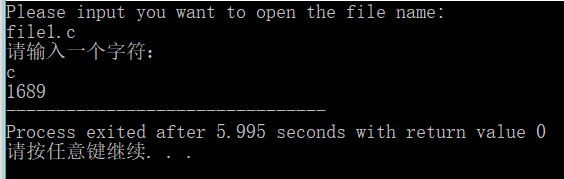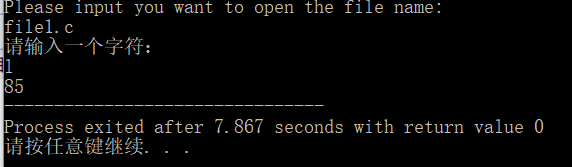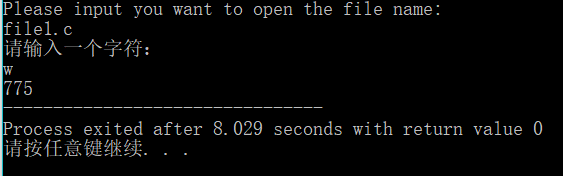### 3.作业所用的知识点：

1.定义文件型指针变量
FILE *fp；
2.打开文件的方式

if((fp=fopen("file1",''r")==NULL){
printf("cannot open file\n");
exit(0);
}


fclose(fp);

3.fputc和fgetc函数
fputc(ch,fp);将字符ch的值输出到fp所指向的文件中去
ch=fgetc(fp);从文件中读取一个字符给ch。
4.feof函数
feof函数判断文件是否结束/判断是否是文件末尾
feof(fp);如果文件结束feof(fp)=1，否则feof(fp)=0.

## 2.学习进度和总结

Git https://gitee.com/tmjl/fourth_pta_operation/tree/master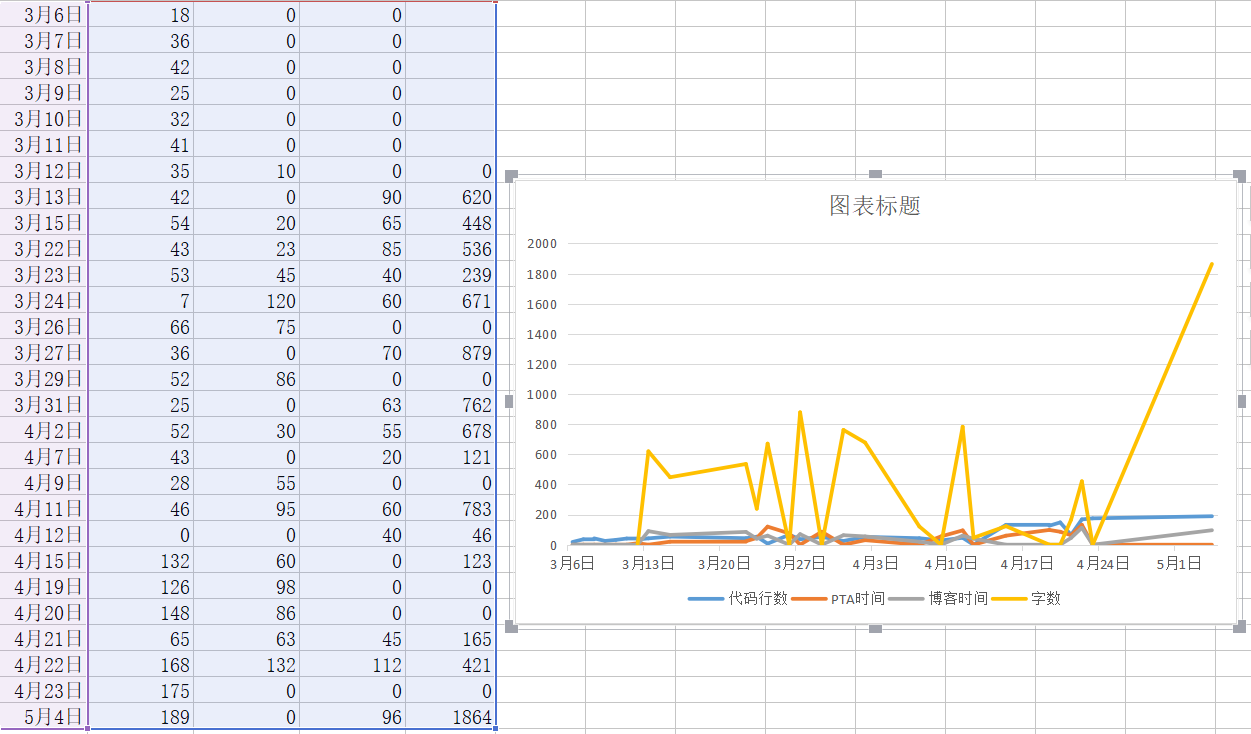### 2.你对目前的上课和作业方式（上课讲解+PTA+博客）有何意见和建议？

PTA作业感觉挺好的，能够及时的巩固上节课所学习的知识点,当做题时遇到不会的知识点能够强迫自己去看书，或者问问同学，能过学到好多有用的东西

### 3.终于我们即将不再是师生。当你结束本课程，你和我就站在同样的位置上，我将不再基于学校授权和知识、经验 (年龄？)上的优势而对你的自由有任何干扰。除发布成绩以外，我最后一次行使教师特权: 请问你有什么要对教师 (我)说的，建议、抱怨、希望……多谢。

posted @ 2018-05-04 22:32  _晨曦  阅读(220)  评论(10编辑  收藏  举报